# Analytical Reasoning Questions for RRB NTPC PDF

0
2565

## Analytical Reasoning Questions for RRB NTPC PDF

Download RRB NTPC Analytical Reasoning Questions and Answers PDF. Top 15 RRB NTPC Analytical Reasoning questions based on asked questions in previous exam papers very important for the Railway NTPC exam.

Instructions

The given Venn diagram shows the categories of people who are Rock Stars, Pop Stars and Hip Stars. Study the diagram carefully and answer the questions that follow: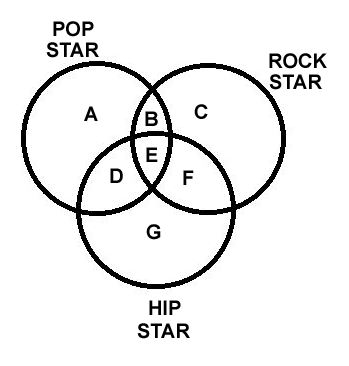Question 1: What is the total number of Rock Stars in the diagram?

a) Only C

b) C + B

c) C+ B + F

d) C+ B + E + F

e) C+B+E+F+ D

Question 2: Which area represents Rock Stars who are Pop Stars but not Hip Stars?

a) A

b) C

c) G

d) B

e) F

Question 3: What does area A represent?

a) Rock Stars who are Pop Stars but not Hip Stars

b) Pop Stars who are neither Rock Stars nor Hip Stars

c) Rock Stars who are Hip Stars but not Pop Stars

d) Hip Stars who are Pop Stars but not Rock Stars

e) Rock Stars who are Pop Stars and Hip Stars

Question 4: What area represents the Hip Stars who are Rock Stars and Pop Stars?

a) A

b) B

c) C

d) D

e) E

Question 5: What does area F represent?

a) Rock Stars who are Pop Stars and Hip Stars

b) Pop Stars who are not Rock Stars and Hip Stars

c) Rock Stars who are Hip Stars but not Pop Stars

d) Hip Stars who are Pop Stars but not Rock Stars

e) Rock Stars who are Pop Stars but not Hip Stars

Question 6: Select the option that fits in the blank space in the given Problem Figure.

a)

b)

c)

d)

Instructions

<p “=””>In each of the questions given below which one of the five answer figures on the right should come after the problem figures on the left, if the sequence were continued?

Question 7: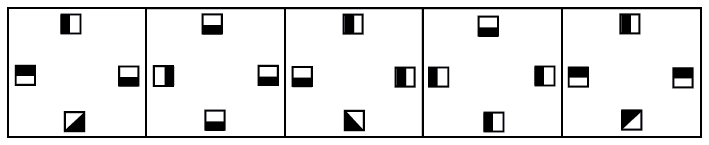a)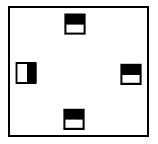b)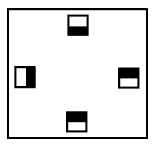c)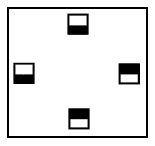d)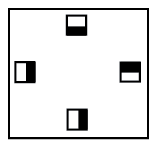e)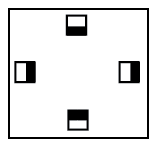Question 8: Which of the following figures represents the relation between ‘Sparrows’, ‘Birds’ and ‘Crows’ ?

a)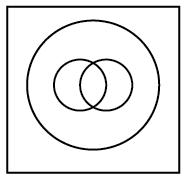b)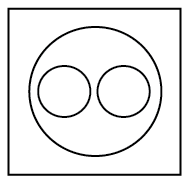c)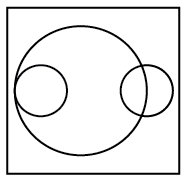d)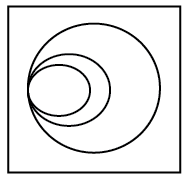e)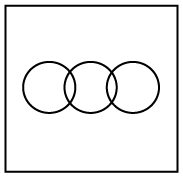Instructions

Find the missing number from the given figures in each of the following questions.

Question 9:a) 36

b) 72

c) 144

d) 18

e) none of these

Question 10:a) 1

b) 2

c) 3

d) 4

e) 5

Question 11: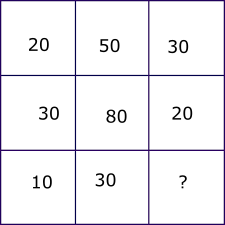a) 20

b) 10

c) 50

d) -10

e) none of these

Question 12:a) 60

b) 140

c) 30

d) 80

e) 90

Question 13: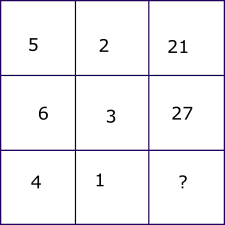a) 15

b) 10

c) 17

d) 20

e) 18

Instructions

Find the missing number from the given figures in each of the following questions.

Question 14:a) 80

b) 100

c) 135

d) 105

e) 120

Instructions

In each of the following questions, there are five problem figures and five answer figures. Problem figures have a pattern. A series is established if one of the answer figures is placed in the question marked space. You have to determine which answer figure best suits the question marked space.

Question 15: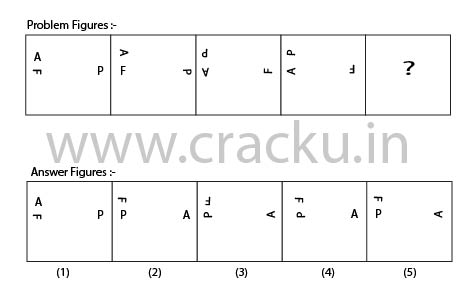a) 1

b) 2

c) 3

d) 4

e) 5

The circle on the top right is entirely Rock Stars. C is only Rock Stars. F is rockstars who are also Hip Stars. B is Rockstars who are also Pop Stars. E is all the three. So, (d) is the answer..

The area of intersection of the two circles at the top is the area of interest. So, the answer is B.

Area A represents “only Popstars”. So, they are neither Rock Stars nor Hip Stars.

The area that represents all the three groups is the area of intersection of the 3 circles ie E.

F is the intersection of right circle and the bottom circle. So, it includes Rock Stars who are Hip Stars but not Pop Stars.

Let’s divide the pattern into 4 portions, left, right, top and bottom.

In the top portion, there is an alternating pattern of the black side of the box, so the black side will be at top in solution figure.

In the left and bottom portion, the black side of the box is rotating in clockwise direction, so the black side will be at right and top side respectively in solution.

In the right portion, the black side of the box is rotating anti clockwise in pairs of 2.

Thus, option (B) satisfies above conditions.

Sparrows and crows are entirely different. But, both are birds.

=> Ans – (B)

The relation between the different terms in a figure is as follows:
Figure 1: (88 x 21)/33 = 56
Figure 2: (65 x 24)/52 = 30
Figure 3: (68 x 45)/? = 85
On solving we get ? = 36

The pattern followed in the figures is as follows:
Figure 1: 6 = (8 + 5) – (5 + 2)
Figure 2: 11 = (9 + 7) – (4 + 1)
Figure 3: 5 = (10 + 6) – (8 + ?)
Thus, ? = 3

The given grid has a vertical relation. The value in the third row is equal to the difference of the first row from the second row.
10 = 30 – 20 and 30 = 80 – 50. In the same way, ? = 20 – 30 = -10

The relation between the terms in each figure is as shown:
Figure 1: (60 – 25)x4 = 140
Figure 2: (45 – 20)x4 = 100
Figure 3: ( 25 – 10)x4 = 60

Figure

The given grid has a horizontal relation. The value in the third column is equal to the difference in squares of the value in the first column and the second column. Thus,
$5^2 – 2^2$ = 25 – 4 = 21 and $6^2 – 3^2$ = 36 – 9 = 27
In the same way, the missing number is equal to $4^2 – 1^2$ = 16 – 1 = 15

Figure 1- $27^{1/3} \times 64^{1/3} \times 1^{1/3}$ = 12
Figure 2 $8^{1/3} \times 216^{1/3} \times 125^{1/3}$ = 60
Figure 3- $125^{1/3} \times 343^{1/3} \times 127^{1/3}$ = 105